# Ballistic curve

The ballistic grenade was fired at a 45° angle. The first half ascended, the second fall. How far and how far it reached if his average speed was 1200km/h, and 12s took from the shot to impact.

Correct result:

x =  0 km
h =  0 m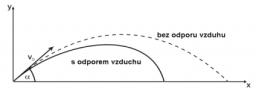We would be pleased if you find an error in the word problem, spelling mistakes, or inaccuracies and send it to us. Thank you!Tips to related online calculators
Our vector sum calculator can add two vectors given by its magnitudes and by included angle.
Do you want to convert velocity (speed) units?

#### You need to know the following knowledge to solve this word math problem:

We encourage you to watch this tutorial video on this math problem:

## Next similar math problems:

• The cruise ship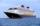The cruise ship has a speed of 12 km/h at a calm surface. When we sail 45 km along the river and 45 km back, it took us exactly 8 hours. Which (constant) speed of flow of the river?
• Ball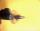The Ball was fired at an angle of 35° at an initial velocity of 292 m/s. Determine the length of the litter. (g = 9.81 m/s2).
• Peleton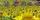Cycling race was run at an average speed of 45 km/h. One cyclist lost with defect 8 minutes. How long and how far he must go at speed 51 km/h to catch peleton again?
• Average speedWhen the bus stops at bus stops driving average speed is 45 km/h. If it did not stop it drive at speed 54 km/h. How many minutes of every hour it spend at stops?
• Resultant forceCalculate mathematically and graphically the resultant of a three forces with a common centre if: F1 = 50 kN α1 = 30° F2 = 40 kN α2 = 45° F3 = 40 kN α3 = 25°
• Two aircraftFrom the airport will start simultaneously two planes, which fly tracks are perpendicular to each other. The first flying speed of 680 km/h and the second 840 km/h. Calculate how far the aircraft will fly for half an hour.
• FighterA military fighter flies at an altitude of 10 km. From the ground position, it was aimed at an altitude angle of 23° and 12 seconds later at an altitude angle of 27°. Calculate the speed of the fighter in km/h.
• The cyclistThe cyclist drove from A to B, 60 km away. The first half drove at 30km/h, the second at 20km/h. How long did it take for him?
• Express train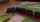International express train drove from Kosice to Teplice. In the first 279 km, the track was repaired, and therefore it was moving at a speed of 10km/h less than it was scheduled to drive. The rest of the 465 km trip has increased the speed by 8 km/h than
• Average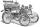What is the average speed of the car, where half of the distance covered passed at speed 66 km/h and the other half at 86 km/h.
• Journey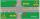Charles and Eva stands in front of his house, Charles went to school south at speed 5.4 km/h, Eva went to the store on a bicycle eastwards at speed 21.6 km/h. How far apart they are after 10 minutes?
• The shooter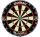The shooter heard the impact of the bullet on the target in one second after the shot. The bullet was moving at an average speed of 500 m/s. Calculate with speed of sound of 340 m/s. Determine the distance of the target.
• Two trains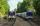Two trains go at each other. First at speed 40 km/h and the second at speed 60 km/h. At what distance will be 30 minutes before they will meet?
• ParatrooperAfter the parachute is opened, the paratrooper drops to the ground at a constant speed of 2 m/s, with the sidewinding at a steady speed of 1.5 m/s. Find: a) the magnitude of its resulting velocity with respect to the ground, b) the distance of his land fr
• MovementFrom the crossing of two perpendicular roads started two cyclists (each at the different road). One runs at average speed 28 km/h, the second at average speed 24 km/h. Determine the distance between them after 45 minutes cycling.
• Two airportsTwo airports are 2690 km away. From the first airport, the airplane flies at a speed of 600 km/h, from a second airplane at a speed of 780 km/h. When will they meet if they flew at 10:00? How far from the first airport?
• Two citiesThe car goes from city A to city B at an average speed of 70 km/h, back at an average speed of 50 km/h. If it goes to B and back at an average speed of 60 km/h, the whole ride would take 8 minutes less. What is the distance between cities A and B?